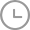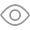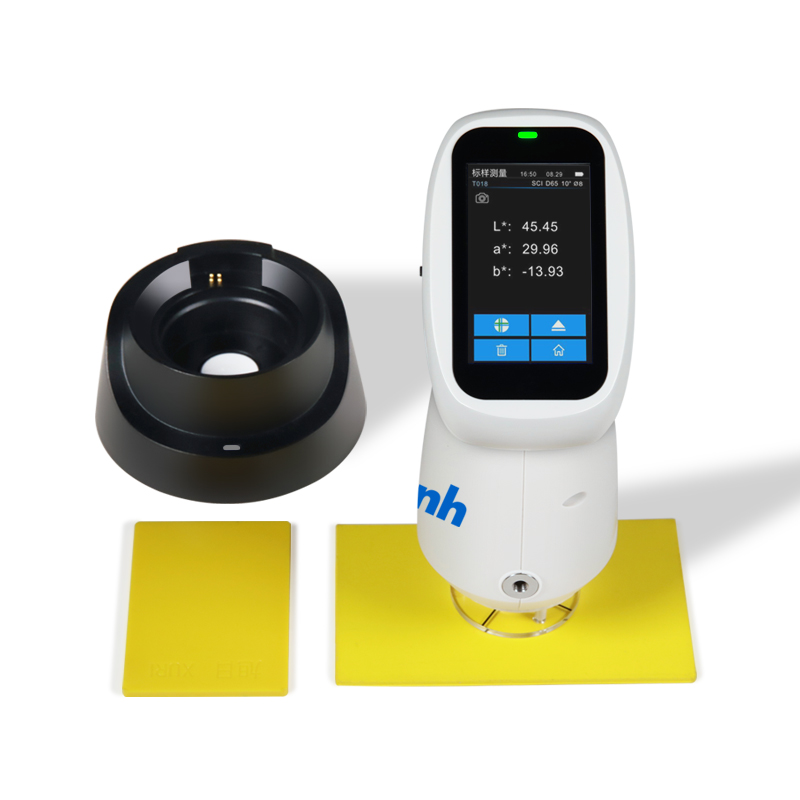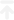-科技创新 真诚服务-

## 售前售后免费技术支持，上门服务# 色差仪上的lab值和XYZ值含义时间：2022-12-23浏览量： 299lab值转换成XYZ值得方法

1. var_Y = ( CIE-L* + 16 ) / 116

2. var_X = CIE-a* / 500 + var_Y

3. var_Z = var_Y - CIE-b* / 200

4. if ( var_Y^636f7079e799bee5baa6313333376138653 > 0.008856 ) var_Y = var_Y^3

5. else                      var_Y = ( var_Y - 16 / 116 ) / 7.787

6. if ( var_X^3 > 0.008856 ) var_X = var_X^3

7. else                      var_X = ( var_X - 16 / 116 ) / 7.787

8. if ( var_Z^3 > 0.008856 ) var_Z = var_Z^3

9. else                      var_Z = ( var_Z - 16 / 116 ) / 7.787

10. X = ref_X * var_X     //ref_X =  95.047     Observer= 2°, Illuminant= D65

11. Y = ref_Y * var_Y     //ref_Y = 100.000

12. Z = ref_Z * var_Z     //ref_Z = 108.883

CIE1976 L*a*b*均匀色空间在涂料、建材、油漆等表面色料工业的配色方面有着广泛的应用,也称为CIELAB均匀色空间。其中L*为明度坐标,表征颜色的亮度大小;a*b*为色品坐标,表征颜色的色调和饱和度,如图：

“L”代表物体的明亮度：0-100表示从黑色到白色

“a”代表物体的红绿色：正值表示红色,负值表示绿色

“b”代表物体的黄蓝色：正值表示黄色,负值表示蓝色

△L=L样品-L标准(明度差异)

△a=a样品-a标准(红/绿差异)

△b=b样品-b标准(黄/蓝差异)

△L+表示偏白,△L-表示偏黑

△a+表示偏红,△a-表示偏绿

△b+表示偏黄,△b-表示偏蓝

△E=[(△L)+(△a)+(△b)]1/2

△L为正值,表示被测品比样品偏亮,为负值表示被测品比样品偏暗;

△A为正值,表示被测品比样品偏红,为负值表示被测品比样品偏绿;

△B为正值,表示被测品比样品偏黄,为负值表示被测品比样品偏蓝;

LCH色空间中, L为亮度, C为饱和度, H 为色调。 亮度,饱和度和色调是颜色的三个基本属性。

RGB 色空间中, R为红色, G为绿色, B为蓝色。这个色空间在印刷行业用的比较多。

XYZ 色空间中, XYZ值是通过物体表面的反射特性和成都来分析颜色的。上一页 : 什么是色彩色差仪?色彩色差仪有哪些应用? 返回 下一页 : 爱色丽分光测色仪使用常见故障及解决方法